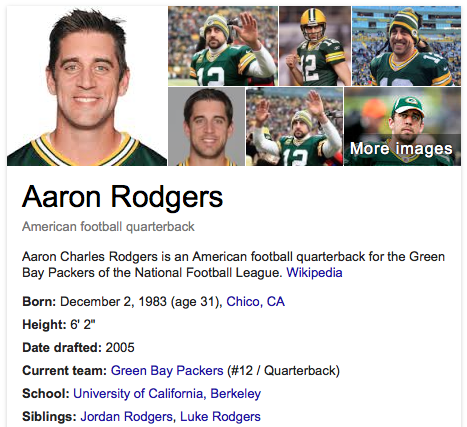## Wednesday, September 30, 2015

### 33 66 | MNF, September 28, 2015, Packers at Chiefs

Notice the sum of MNF, 38+28 = 66.

MNF = 13+14+6 = 33
G = 33 (Francis Bacon Gematria)
Thirty-Three = 2+8+9+9+2+7+2+8+9+5+5 = 66

This year's upcoming Super Bowl is 50, or 'Fifty'.

Fifty = 6+9+6+20+25 = 66

Notice the date of Super Bowl I.

1/15/67 = 1+15+67 = 83
Football = 6+15+15+20+2+1+12+12 = 83

The first Super Bowl was in the 47th Season of the NFL, at the close of '66.  It is interesting to note that the 49ers joined the NFL in 1949, and have been in the league for 66-years this season.  Again, they are hosting Super Bowl 50, which has the connection to '66'.

Mike McCarthy's brother died at age 47 earlier this year.Chico, CA = 3+8+9+3+6+3+1 = 33 (Also, CC initials, another 33)

Notice Aaron Rodger's birthday, December 2, or 12/2.  This has a connection to 'San Francisco'.

12/2/1983 = 1+2+2+1+9+8+3 = 26
12/2/1983 = 12+2+(1+9+8+3) = 35
12/2/1983 = 12+2+19+83 = 116
12/2/83 = 12+2+83 = 97

Aaron = 1+1+9+6+5 = 22
Charles = 3+8+1+9+3+5+1 = 30/39
Rodgers = 9+6+4+7+5+9+1 = 41/50
Aaron Charles Rodgers = 93/111

In his MNF performance, Rodgers had 35 attempts for 333 passing yards.  In the game, the Packers ended the Chiefs undefeated record at Lambeau.  This is extra interesting because Aaron Rodgers defeated Alex Smith, the Quarterback who was drafted #1 overall in the 2005 NFL Draft, by the San Francisco 49ers, ahead of him.  For Aaron Roders, this was a major disappointment at the time because he had left Cal early in hopes of being taken #1 by the 49ers, his favorite NFL team.  Rodgers said that he wore a Joe Montana jersey under his suit for the draft.

Alex Smith was #1, Aaron Rodgers was #24.  https://en.wikipedia.org/wiki/2005_NFL_draft

An interesting note, Alex Smith threw 1 TD and 11 INTs in his rookie season.

Alex = 1+12+5+24 = 42
Smith = 19+13+9+20+8 = 69
Alex Smith = 111 (1 TD, 11 INTs...) (#1 pick...)

Interesting to think that both he and Rodgers have connections to the number '111'.

#### 1 comment:

1.Also the first Superbowl was between, Green Bay and Kansas City!!